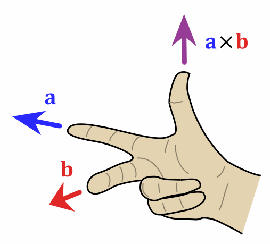The picture to the right shows a hand that illustrates how the cross product of two vectors (a and b) generate a vector that is perpendicular to both a and b. Now try to imagine four vectors that are all perpendicular to each other. This is kind of tricky, mainly because it is impossible for four 3-dimensional vectors to all be perpendicular to each other.

But it is not impossible if the vectors are 4-dimensional, however that creates another problem: it is (at least for me) not possible to mentally picture a 4-dimensional space. Luckily I don’t have to the math works anyway :) The reason I post this is that I am porting an old 4D Julia Raytracer from C++ to C# and was just struck by this magic function:

```/// <summary>
/// Calculates a quaternion that is perpendicular to three other quaternions.
/// Quaternions are handled as vectors in 4d space
/// </summary>
public static Quaternion Cross4D(Quaternion q1,Quaternion q2,Quaternion q3)
{
double b1c4=q2.r*q3.k-q2.k*q3.r;
double b1c2=q2.r*q3.i-q2.i*q3.r;
double b1c3=q2.r*q3.j-q2.j*q3.r;
double b2c3=q2.i*q3.j-q2.j*q3.i;
double b2c4=q2.i*q3.k-q2.k*q3.i;
double b3c4=q2.j*q3.k-q2.k*q3.j;

var r = -q1.i*b3c4+q1.j*b2c4-q1.k*b2c3;
var i =  q1.r*b3c4-q1.j*b1c4+q1.k*b1c3;
var j = -q1.r*b2c4+q1.i*b1c4-q1.k*b1c2;
var k =  q1.r*b2c3-q1.i*b1c3+q1.j*b1c2;

return new Quaternion(r, i, j, k);
}```

This is a function that calculates a cross product for a 4-dimensional vector (the Quaternion class is used as a 4-dimensional vector in imaginary space). The reason for the naming of variables in the calculation relates to how the cross product formula is derived (as the determinant of a matrix). Anyway, I just found it funny that no matter how hard I try I cannot picture what this function actually generates. This is probably nothing new for mathematicians or physicist who I guess daily has to fight against the limitations of the human mind.

But the math works, I can position the camera in 4D space and render pictures of the 4-dimensional Julia Set :)

On a side note this app was MUCH easier to parallelize (using Parallel.For from the Parallel Extensions Library) than GenArt. Because the algorithm works like a raytracer the outer ray casting loop is easily implemented using Parallel.For which instantly gave a 4x performance increase on my quad core CPU.

I took some time to upload some of the old animations to youtube, they were rendered many years ago using the C++ version.

Here is an animation of a camera move around the Julia set, the camera is moving in the second (i) and fourth (k) imaginary dimension.

Here is another one, which I really like, the Julia constant is moving in a small circle in the first and second dimension. It gives me a strange impression of something organic and fluid. When I and my friend presented this rendering technique the first slide had this animation in a loop :)

You can see an interesting artifact of the rendering algorithm in the video above. The Julia Set actually hits the camera plane. The camera has a near plane where we start traversing the rays and a far plane where we stop, what happens is that the middle expands beyond the near plane, creating a flat surface.